TOTAL LAG TIME

Single Line Application
By: Glenn Sheffield

Total lag time for a sample loop is the total internal volume of the transport tubing and conditioning devices divided by the flow rate in consistent units as follows:This equation should be modified to allow for first order lag and a compressibility factor for a gas sample. First order lag is the amount of time required to displace enough volume through a sample-conditioning device to ensure the sample is representative of a process change i.e., mixing time. This is generally a factor of 3 times the volume.

The compressibility factor for gas reflects the relative density of molecules in a given volume, which is proportional to the pressure of the sample. Taking the absolute pressure of the sample gas and dividing by 14.7 PSIA calculate the compressibility factor. Thus the higher the pressure of a gas sample the greater the time required to transport it to the analyzer or to evacuate the sample from a conditioning device.

The temperature factor is not included for simplicity and because it has a negligible effect in the temperature range of most field applications.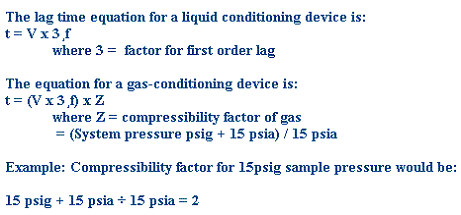VOLUME CALCULATIONS
TRANSPORT LINE
Calculating the volume of transport tubing is simplified by using the known volumes of common tubing. The volumes of standard size tubes are as follows: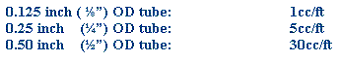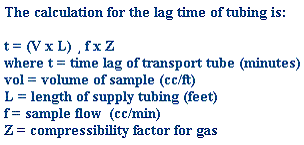SAMPLE CONDITIONING DEVICE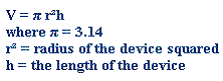WORKING EXAMPLE
An analyzer has a single line sample transport system with 100ft. of ¼” tubing from a regulator set at 15psig. Due to the relatively clean sample the only sample-conditioning device is a 3 inch high x 2.5inch wide filter. The flow to the analyzer and bypass rotometer is a total of 1000 cc/min (2 scfh).
What is the lag time of the transport system? What is the lag time of the filter housing? What is the total lag time? Is this considered excessive?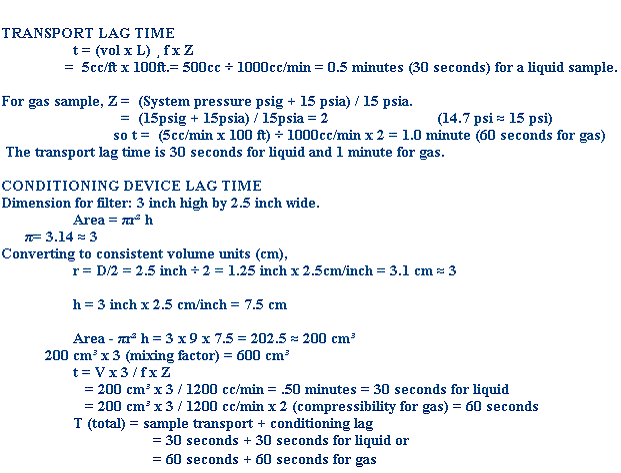The rule of thumb is a maximum of 1 minute for transport and 30 second conditioning time. The following is a comparison of the common filter used for the example above and the Sheffield kinetic separator. The same flow rates and pressures are assumed and thus only the dimensions are changed.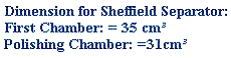No mixing lag is necessary because the narrow dimensions of the chambers allows the separator to displace sample at a rate similar to tubing which does not requires mixing lag.

SS1200

First Chamber: 35cc/1200cc/min.= .029 min. = 1.75 seconds for liquid

Polishing Chamber: 31cc/1000cc/min. = .031min. = 1.86 seconds for liquid

Total Lag Time for Liquid = 3.61 seconds

Total Lag Time for Gas = 3.61 x 2 (Comp. Factor) = 7.22 seconds

SS700

First Chamber: 20cm / 1200 cc/min = .016 min = 1 seconds x 2 First Oder Lag = 2 seconds

Polishing Chamber: 20cm / 1000 cc/min. = .02 min. = 1.2 seconds x 2 First Oder Lag = 2.4

Total Lag Time for Liquid = 4.4 seconds.

Total Lag Time for Gas = 2 x 4.4 seconds (Comp. Factor) = 8.8 seconds for Gas.

SS300

First Chamber: 7cm / 1200 cc/min = .005 min = .3 seconds x 3 First Oder Lag = .9 seconds

Polishing Chamber: 7cm / 1000 cc/min. = .007 min. = .42 seconds x 3 First Oder Lag = 1.26 seconds

Total Lag Time for Liquid = 2.16 seconds.

Total Lag Time for Gas = 2 x 2.16 seconds (Comp. Factor) = 4.3 seconds for Gas

Plug in your numbers in the table below.

Lag Time Chart 915